## Example5.2 pagenumber 231¶

In :
#Given
L_ab = 0.4  #mt The total length of the rod
M = 200     #N_m - the moment acting on rod
l_1 = 0.1   #mt -moment acting point the distance from 'a'
R_1 = 100   #N - The Force acting
l_2 = 0.2   #mt -R_1 acting point the distance from 'a'
R_2 = 160   #N The Force acting
l_3 = 0.3   #mt -R_2 acting point the distance from 'a'
#caliculations

#F_X = 0 forces in x directions
R_A_X = 0   # since there are no forces in X-direction
R_B_X = 0
#M_A = 0 momentum at point a is zero

# M + R_1*l_2 + R_2*l_3 = R_B*L_ab *the moment for a force is FxL
R_B_Y =  (M + R_1*l_2 + R_2*l_3)/L_ab

#M_B= 0 momentum at point b is zero
# R_A_Y*L_ab + M - R_1*l_2 - R_2*0.1 = 0

R_A_Y = -(M - R_1*l_2 - R_2*0.1)/L_ab

print "The X,Y components of reaction force at A is ",R_A_X,",",R_A_Y,"N"
print "The X,Y components of reaction force at B is ",R_B_X,",",R_B_Y,"N"

The X,Y components of reaction force at A is  0 , -410.0 N
The X,Y components of reaction force at B is  0 , 670.0 N


## Example 5.2 page number 233¶

In :
#Given
P_Max  = 10   #N - the maximum distribution in a triangular distribution
L = 3         #mt the total length of force distribution
L_X = 5       #mt - the horizantal length of the rod
#caliculations

F_y = P_Max*L*0.5 #N - The force due to triangular distribition
L_com  = 2*L /3   #mt - the resultant force acting as a result of distribution acting position
#F_X = 0 forces in x directions
R_A_X = 0         # since there are no forces in X-direction
R_B_X = 0
#M_A = 0 momentum at point a is zero
#F_y*L_com - R_B_Y*L_X = 0
R_B_Y = F_y*L_com/L_X

#M_B= 0 momentum at point b is zero
#- R_A_Y*L_X = F_y*(L_X-L )

R_A_Y = - F_y*L/L_X
print "The X,Y components of reaction force at A is ",R_A_X,",",R_A_Y,"N"
print "The X,Y components of reaction force at B is ",R_B_X,",",R_B_Y,"N"

The X,Y components of reaction force at A is  0 , -9.0 N
The X,Y components of reaction force at B is  0 , 6.0 N


## Example 5.3 page number 233¶

In :
#given
F = 5       #K - force acting on the system
tan = (4/3) # the Tan of the angle of force with x axis
l_ab = 12   #inch - the total length of ab
l = 3       # inch - Distance from 'a'
#caliculation
F_X = 4 #K
F_Y = 3 #k

#M_A = 0 momentum at point a is zero
# F_X*l- R_B_Y*l_ab = 0
R_B_Y = F_X*l/l_ab

#M_B= 0 momentum at point b is zero
# R_A_Y*l_ab - F_X*(l_ab - l)
R_A_Y = F_X*(l_ab - l)/l_ab

#F_X = 0 forces in x directions
R_A_X = F_Y + R_B_Y
R_B_X = R_B_Y      # since the angle is 45 degrees

#resultants
R_A = pow(R_A_X**2 + R_A_Y**2,0.5)
R_B = pow(R_B_X**2 + R_B_Y**2,0.5)

print "The X,Y components and resultant of reaction force at A is ",R_A_X,",",R_A_Y,",",R_A,"N"
print "The X,Y components and resultant of reaction force at B is ",R_B_X,",",R_B_Y,",",round(R_B,2),"N"

The X,Y components and resultant of reaction force at A is  4 , 3 , 5.0 N
The X,Y components and resultant of reaction force at B is  1 , 1 , 1.41 N


## Example 5.4 page number 239¶

In :
#Given
P_Max  = 10   #N - the maximum distribution in a triangular distribution
L = 3         #mt the total length of force distribution
L_X = 5       #mt - the horizantal length of the rod
#caliculations

F_y = P_Max*L*0.5 #N - The force due to triangular distribition
L_com  = 2*L /3   #mt - the resultant force acting as a result of distribution acting position
#F_X = 0 forces in x directions
R_A_X = 0         # since there are no forces in X-direction
R_B_X = 0
#M_A = 0 momentum at point a is zero
#F_y*L_com - R_B_Y*L_X = 0
R_B_Y = F_y*L_com/L_X

#M_B= 0 momentum at point b is zero
#- R_A_Y*L_X = F_y*(L_X-L )

R_A_Y = - F_y*L/L_X

#For a---a section
l_a = 2 #mt - a---a section from a
l_com_a = 2*l_a/3
v_a = R_A_Y + 0.5*l_a*(10.0*2/3) #*(10*2/3) because the maximum moves

M_a =  (10.0*0.66)*l_a*(0.33) + R_A_Y*l_a

#For b---b section

v_b = F_y + R_A_Y #equilabrium conditions
M_b =  (F_y + R_A_Y)*(-1)

print "The force and moment in section a--a are",round(v_a,2),"KN ,",M_a,"KN-m"
print "The force and moment in section b--b are",v_b,"KN ,",M_b,"KN-m"

The force and moment in section a--a are -2.33 KN , -13.644 KN-m
The force and moment in section b--b are 6.0 KN , -6.0 KN-m


## Example 5.5 page number 241¶

In :
#Given
#Lets divide the section into two sections
l_ac = 10 # ft -The total length of the rod
R = 5     #k - The applies force at c
tan = 4/3 # The tan of the angle of the force
l_ab = 5  #ft - The distance of applied force from A
R_y = 4 #k,downwards X- component of the force
R_X = 3 #k Y- component of the force , lets consider only Y direction since we are concentrating on the Shears

#F_Y = 0 forces in Y directions
#R_A +R_B =  R_y
#M_c = 0 making the moment zero at point c
#Caliculations
# R_A= R_B*(l_ac - l_ab)/(l_ab) so R_A = R_B

R_A =  R_y/2 #F_Y = 0
R_B =  R_y/2
#considering section x--x
l_x = 2       #ft - length of section from A
v_x = R_A     #k ,F_X = 0
M_x = R_A*l_x #k-ft M_c = 0

#considering section at midpoint t--t
l_t =  2        #ft - length of section from A
v_t = 0         #k ,F_X = 0
M_t = (R_A)*l_t #k-ft M_c = 0

##considering section y---y
l_y = 2       #ft - length of section from B
v_y = - R_B   #k ,F_X = 0
M_y = R_B*l_y #k-ft M_c = 0

#Graph
%matplotlib inline
import math
from matplotlib.pyplot import plot,suptitle,xlabel,ylabel
#Drawing of shear and bending moment diagram
print "Given problem is for drawing diagram, this diagram is drawn by step by step manner. "
X = [0,2,4.9999999999999,5,5.00000000000000001,7,10] # For graph precision

V = [R_A,v_x,v_x,v_t,v_y,v_y,-R_B];			#Shear matrix
M = [0,M_x,M_t,M_t,M_t,M_y,0];			#Bending moment matrix
plot(X,V);			#Shear diagram
plot(X,M,'r');			#Bending moment diagram
suptitle( 'Shear and bending moment diagram')
xlabel('X axis')
ylabel( 'Y axis') ;

Given problem is for drawing diagram, this diagram is drawn by step by step manner.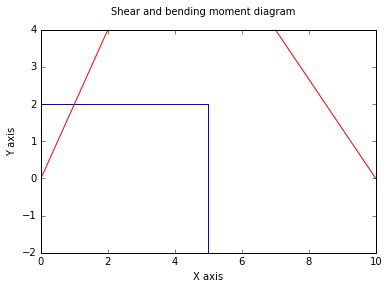## Example 5.6 pagenumber 243¶

In :
#Given
l = 1                       #L - Length of the cantilever
F_app = ((2**0.5))*2        #p - force applies
tan = 1                     # The angle of force applied
F_app_x = F_app/((2**0.5))  #p The horizantal component of the force , neglected
F_app_y = F_app/((2**0.5))  #p  The Vertical component of the force
#F_Y = 0
R_A = 1 #p

#Considering  section 1-----1
l_1 = 0.5       # The length of the section from one end
v_1 = R_A       #F_Y = 0
M_1 = -R_A*l_1  #MAking moment at section 1 = 0

#considering end of cantilever
l_2 = 1       # The length of the section from one end
v_2 = R_A     #F_Y = 0
M_2 = -R_A*l_2#MAking moment at section 1 = 0

#Graph
%matplotlib inline
import math
from matplotlib.pyplot import plot,suptitle,xlabel,ylabel
#Drawing of shear and bending moment diagram
print "Given problem is for drawing diagram, this diagram is drawn by step by step manner. "
X = [0,0.5,1] # For graph precision

V = [R_A,v_1,v_2 ];			#Shear matrix
M = [0,M_1,M_2];			#Bending moment matrix
plot(X,V);			        #Shear diagram
plot(X,M,'r');			    #Bending moment diagram
suptitle( 'Shear and bending moment diagram')
xlabel('X axis')
ylabel( 'Y axis') ;

Given problem is for drawing diagram, this diagram is drawn by step by step manner.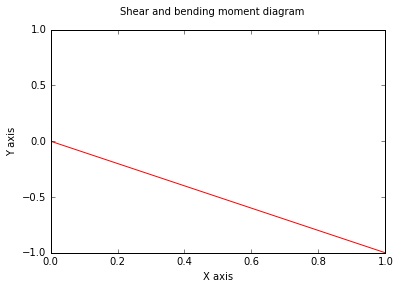## Example 5.7 page number 243¶

In :
#Given
l_ab = 3  #L - The total length lets say '3L'
R_1 = 1   #p - The force applied at b
R_2 = 1   #p - The force applied at c
l_ab = 1  #L
l_bc = 1  #L

#Logical step
#Since the system is in symmetry we can avoid moment M = 0 caliculations

#F_Y = 0
R_A = (R_1 + R_2)/2
R_B = (R_1 + R_2)/2

#Lets take '3' sections
#Considering section 1-----1 at 0.5L
l_1 = 0.5     #L - distance of the section from the A
v_1 = R_A     #F_Y = 0
M_1 = R_A*l_1 #MAking moment at section 1 = 0

#Considering section 2-----2 at 1L
l_2 = 1       #L - distance of the section from the A
v_2 = R_A     #F_Y = 0
M_2 = R_A*l_2 #MAking moment at section 2 = 0

#Considering section 3-----3 at 1.5L
l_3 = 1.5       #L - distance of the section from the A
v_3 = 0        #F_Y = 0
M_3 = R_A*l_2  #MAking moment at section 2 = 0 and symmetry

#GRAPH
#Since the symmetry exists the graphs are also symmetry
%matplotlib inline
import math
from matplotlib.pyplot import plot,suptitle,xlabel,ylabel
#Drawing of shear and bending moment diagram
print "Given problem is for drawing diagram, this diagram is drawn by step by step manner. "
X = [0,0.5,1,1.000000001,1.5,1.999999999999,2,2.5,3] # For graph precision

V = [R_A,v_1,v_2,v_3,v_3,v_3,-v_2,-v_1,-R_B];			#Shear matrix
M = [0,M_1,M_2,1,1,1,M_2,M_1,0];			#Bending moment matrix
plot(X,V);			#Shear diagram
plot(X,M);			#Bending moment diagram
suptitle( 'Shear and bending moment diagram')
xlabel('X axis')
ylabel( 'Y axis') ;

Given problem is for drawing diagram, this diagram is drawn by step by step manner.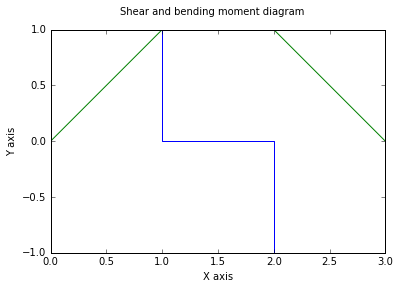## Example 5.8 page nmber 244¶

In :
#Given
%matplotlib inline
import numpy as np
l_ab = 1.0   #L - The length of the beam
F_D = 1.0    #W - The force distribution
F = F_D*l_ab #WL - The force applied
#Beause of symmetry the moment caliculations can be neglected
#F_Y = 0
R_A = F/2 #wl - The reactive force at A
R_B = F/2 #wl - The reactive force at B

#considering many sections

#section 1--1
l_1 = [0,0.1,0.2,0.3,0.4,0.5,0.6,0.7,0.8,0.9,1] #L taking each section at 0.1L distance
M_1 = [0,0,0,0,0,0,0,0,0,0,0]
v = [0,0,0,0,0,0,0,0,0,0,0]
for i in range(10):
v[i] = R_A - F_D*l_1[i]
M_1[i] = R_A*l_1[i] - F_D*(l_1[i]**2)/2 #M = 0 in the section
print R_A
#Graphs
import numpy as np
values = [0.5,0,-0.5]
y = np.array(values)
t = np.linspace(0,1,3)
poly_coeff = np.polyfit(t, y, 2)
import matplotlib.pyplot as plt
plt.plot(t, y, 'o')
plt.plot(t, np.poly1d(poly_coeff)(t), '-')
plt.show()

import numpy as np
values = M_1
y = np.array(values)
t = np.linspace(0,1,11)
poly_coeff = np.polyfit(t, y, 2)
import matplotlib.pyplot as plt
plt.plot(t, y, 'o')
plt.plot(t, np.poly1d(poly_coeff)(t), '-')
plt.show()

0.5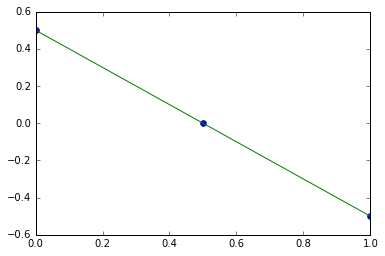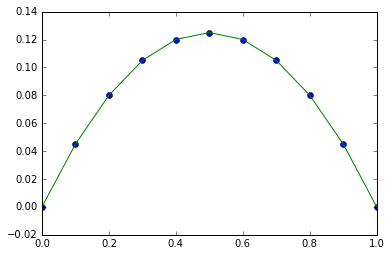## Example 5.9 page number 245¶

In :
#Given
P_Max  = 10   #N - the maximum distribution in a triangular distribution
L = 3         #mt the total length of force distribution
L_X = 5       #mt - the horizantal length of the rod
#caliculations

F_y = P_Max*L*0.5 #N - The force due to triangular distribition
L_com  = 2*L /3   #mt - the resultant force acting as a result of distribution acting position
#F_X = 0 forces in x directions
R_A_X = 0         # since there are no forces in X-direction
R_B_X = 0
#M_A = 0 momentum at point a is zero
#F_y*L_com - R_B_Y*L_X = 0
R_B_Y = F_y*L_com/L_X

#M_B= 0 momentum at point b is zero
#- R_A_Y*L_X = F_y*(L_X-L )

R_A_Y = - F_y*L/L_X

#caliculating for some random value
#For a---a section
l_a = 2 #mt - a---a section from a
l_com_a = 2*l_a/3
v_a = R_A_Y + 0.5*l_a*(10.0*2/3) #*(10*2/3) because the maximum moves

M_a =  (10.0*0.66)*l_a*(0.33) + R_A_Y*l_a

print "The force and moment in section a--a are",round(v_a,2),"KN ,",M_a,"KN-m"

The force and moment in section a--a are -2.33 KN , -13.644 KN-m


## Example 5.13 page number 254¶

In :
#Given
l_ab = 4  #L - The total length lets say '3L'
R_1 = 1   #p - The force applied at b
R_2 = 1   #p - The force applied at c
l_ab = 1  #L
l_bc = 3 #L

#Logical step
#Since the system is in symmetry we can avoid moment M = 0 caliculations

#F_Y = 0
R_A = (R_1 + R_2)/2
R_B = (R_1 + R_2)/2

#Lets take '3' sections
#Considering section 1-----1 at 0.5L
l_1 = 0.5    #L - distance of the section from the A
v_1 = R_A     #F_Y = 0
M_1 = R_A*l_1 #MAking moment at section 1 = 0

#Considering section 2-----2 at 1L
l_2 = 1       #L - distance of the section from the A
v_2 = R_A     #F_Y = 0
M_2 = R_A*l_2 #MAking moment at section 2 = 0

#Considering section 3-----3 at 1.5L
l_3 = 3       #L - distance of the section from the A
v_3 = 0        #F_Y = 0
M_3 = R_A*l_2  #MAking moment at section 2 = 0 and symmetry

#GRAPH
#Since the symmetry exists the graphs are also symmetry
%matplotlib inline
import math
from matplotlib.pyplot import plot,suptitle,xlabel,ylabel
#Drawing of shear and bending moment diagram
print "Given problem is for drawing diagram, this diagram is drawn by step by step manner. "
X = [0,0.5,1,1.0000001,2,2.9999999999,3,3.5,4] # For graph precision

V = [R_A,v_1,v_2,v_3,v_3,v_3,-v_2,-v_1,-R_B];			#Shear matrix
M = [0,M_1,M_2,M_3,M_3,M_3,M_2,M_1,0];			#Bending moment matrix
plot(X,V);			#Shear diagram
plot(X,M);			#Bending moment diagram
suptitle( 'Shear and bending moment diagram')
xlabel('X axis')
ylabel( 'Y axis') ;

Given problem is for drawing diagram, this diagram is drawn by step by step manner.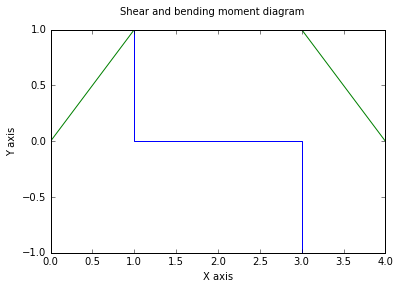## Example 5.14 page number 255¶

In :
#Given
import numpy as np
import matplotlib.pyplot as plt
l = 1.0 #l - The length of the beam
p = 1.0 #W - The total load applied
#since it is triangular distribution
l_com = 0.66*l#l - The distance of force of action from one end
#F_Y = 0
#R_A + R_B = p
#M_a = 0 Implies that R_B = 2*R_A
R_A = p/3.0
R_B = 2.0*p/3

#Taking Many sections

#Section 1----1
l = [0,0.1,0.2,0.3,0.4,0.5,0.6,0.7,0.8,0.9,1] #L taking each section at 0.1L distance
M = [0,0,0,0,0,0,0,0,0,0,0]
v = [0,0,0,0,0,0,0,0,0,0,0]
for i in range(10):
v[i] = p*(l[i]**2) - p/3.0
M[i] = p*(l[i]**3)/(3.0)- p*l[i]/3.0

v = R_B #again concluded Because the value is tearing of

#Graph
values = M
y = np.array(values)
t = np.linspace(0,1,11)
poly_coeff = np.polyfit(t, y, 2)

plt.plot(t, y, 'o')
plt.plot(t, np.poly1d(poly_coeff)(t), '-')
plt.show()
%matplotlib inline
values = v
y = np.array(values)
t = np.linspace(0,1,11)
poly_coeff = np.polyfit(t, y, 2)

plt.plot(t, y, 'o')
plt.plot(t, np.poly1d(poly_coeff)(t), '-')
plt.show()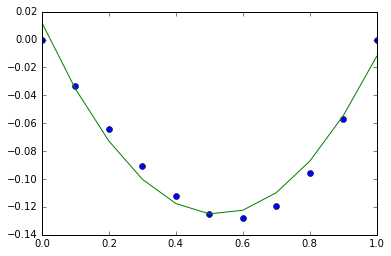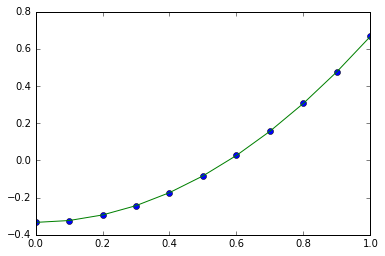## Example 5.16 page number 259¶

In :
#Given
import math
from matplotlib.pyplot import plot,suptitle,xlabel,ylabel
l = 6.0      #a - length of the rod
F = 1.0      #p - force applies in x direction
d = 1.0      #a
M = 1.0      #pa - torque applies on the rod
l_ab = 4.0   #a application of torque point from A
#M = 0 implies that
R_A =  F/6.0 #p - The reaction at A
R_B = - R_A  #F_Y = 0

#Caliculations

#Taking sections
#Section 1---1
l_1 = 1 #a - the length of the section
M_1 = - R_A*l_1  #M = 0

#Section 2---2
l_2 = 4 #a - the length of the section
M_2 = - R_A*l_2  #M = 0

l_4 = 2 #a - the length of the section
M_4 = 1/3.0 #pa #M = 0 '-M' because there is moment couple in between

#Section 3---3
l_3 = 1 #a - the length of the section
M_3 = 1/6.0#pa M = 0 '-M' because there is moment couple in between
print R_A

#GRAPH
#Since the symmetry exists the graphs are also symmetry
%matplotlib inline
#Drawing of shear and bending moment diagram
print "Given problem is for drawing diagram, this diagram is drawn by step by step manner. "
X = [0,1,4,4.00001,5,6] # For graph precision
M = [0,M_1,M_2,M_4,M_3,0];			#Bending moment matrix
plot(X,M);			#Bending moment diagram
suptitle( 'Shear and bending moment diagram')
xlabel('X axis')
ylabel( 'Y axis') ;

0.166666666667
Given problem is for drawing diagram, this diagram is drawn by step by step manner.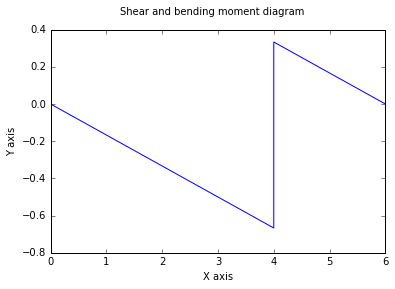In [ ]: